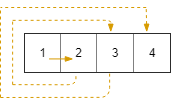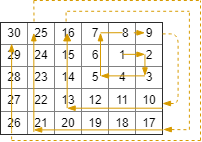##### Welcome to Subscribe On Youtube

Formatted question description: https://leetcode.ca/all/885.html

# 885. Spiral Matrix III

Medium

## Description

On a 2 dimensional grid with R rows and C columns, we start at (r0, c0) facing east.

Here, the north-west corner of the grid is at the first row and column, and the south-east corner of the grid is at the last row and column.

Now, we walk in a clockwise spiral shape to visit every position in this grid.

Whenever we would move outside the boundary of the grid, we continue our walk outside the grid (but may return to the grid boundary later.)

Eventually, we reach all R * C spaces of the grid.

Return a list of coordinates representing the positions of the grid in the order they were visited.

Example 1:

Input: R = 1, C = 4, r0 = 0, c0 = 0

Output: [[0,0],[0,1],[0,2],[0,3]]Example 2:

Input: R = 5, C = 6, r0 = 1, c0 = 4

Output: [[1,4],[1,5],[2,5],[2,4],[2,3],[1,3],[0,3],[0,4],[0,5],[3,5],[3,4],[3,3],[3,2],[2,2],[1,2],[0,2],[4,5],[4,4],[4,3],[4,2],[4,1],[3,1],[2,1],[1,1],[0,1],[4,0],[3,0],[2,0],[1,0],[0,0]]Note:

1. 1 <= R <= 100
2. 1 <= C <= 100
3. 0 <= r0 < R
4. 0 <= c0 < C

## Solution

Starting from (r0, c0), visit the cells and store the visited cells’ rows and columns. How many cells are visited each time in one direction? After visiting (r0, c0), move east by 1 step, and then move south by 1 step. After the two directions, move west by 2 steps, and then move north by 2 steps. It can be seen that the number of steps in each direction increases by 1 for every two directions. In this way, there won’t be any cell that is visited more than once. Continue visiting the cells in this way.

It is possible that a coordinate is out of the boundary of the grid. To store the visited cell’s rows and columns, only store the cells that are inside the boundary of the grid. The visiting procedure ends when all cells inside the boundary of the grid are visited.

• class Solution {
public int[][] spiralMatrixIII(int R, int C, int r0, int c0) {
int cells = R * C;
int[][] order = new int[cells];
order = r0;
order = c0;
int count = 1, fillCount = 1;
int[] currentCell = new int;
currentCell = r0;
currentCell = c0;
int[][] directions = { {0, 1}, {1, 0}, {0, -1}, {-1, 0} };
while (fillCount < cells) {
int step = (count + 1) / 2;
int[] direction = directions[(count - 1) % 4];
for (int i = 0; i < step; i++) {
for (int j = 0; j < 2; j++)
currentCell[j] += direction[j];
if (currentCell >= 0 && currentCell < R && currentCell >= 0 && currentCell < C) {
for (int j = 0; j < 2; j++)
order[fillCount][j] = currentCell[j];
fillCount++;
}
}
count++;
}
return order;
}
}

############

class Solution {
public int[][] spiralMatrixIII(int rows, int cols, int rStart, int cStart) {
int cnt = rows * cols;
int[][] ans = new int[cnt];
ans = new int[] {rStart, cStart};
if (cnt == 1) {
return ans;
}
for (int k = 1, idx = 1;; k += 2) {
int[][] dirs = new int[][] { {0, 1, k}, {1, 0, k}, {0, -1, k + 1}, {-1, 0, k + 1}};
for (int[] dir : dirs) {
int r = dir, c = dir, dk = dir;
while (dk-- > 0) {
rStart += r;
cStart += c;
if (rStart >= 0 && rStart < rows && cStart >= 0 && cStart < cols) {
ans[idx++] = new int[] {rStart, cStart};
if (idx == cnt) {
return ans;
}
}
}
}
}
}
}

• // OJ: https://leetcode.com/problems/spiral-matrix-iii/
// Time: O(max(R, C)^2)
// Space: O(1)
class Solution {
private:
vector<vector<int>> ans;
int r, c;
void add(int x, int y) {
if (x < 0 || x >= r || y < 0 || y >= c) return;
ans.push_back({ x, y });
}
public:
vector<vector<int>> spiralMatrixIII(int R, int C, int r0, int c0) {
r = R;
c = C;
int x = r0, y = c0, cnt = R * C, d = 2;
ans.push_back({ x, y });
while (ans.size() < cnt) {
for (int i = 1; i < d; ++i) add(++x, y);
for (int i = 0; i < d; ++i) add(x, --y);
for (int i = 0; i < d; ++i) add(--x, y);
for (int i = 0; i < d; ++i) add(x, ++y);
d += 2;
}
return ans;
}
};

• class Solution:
def spiralMatrixIII(
self, rows: int, cols: int, rStart: int, cStart: int
) -> List[List[int]]:
ans = [[rStart, cStart]]
if rows * cols == 1:
return ans
k = 1
while True:
for dr, dc, dk in [[0, 1, k], [1, 0, k], [0, -1, k + 1], [-1, 0, k + 1]]:
for _ in range(dk):
rStart += dr
cStart += dc
if 0 <= rStart < rows and 0 <= cStart < cols:
ans.append([rStart, cStart])
if len(ans) == rows * cols:
return ans
k += 2

############

class Solution(object):
def spiralMatrixIII(self, R, C, r0, c0):
"""
:type R: int
:type C: int
:type r0: int
:type c0: int
:rtype: List[List[int]]
"""
def nxt(r, c):
step = 1
yield (r, c)
while True:
for _ in range(step):
c += 1
yield (r, c)
for _ in range(step):
r += 1
yield (r, c)
step += 1
for _ in range(step):
c -= 1
yield (r, c)
for _ in range(step):
r -= 1
yield (r, c)
step += 1
ans = []
for r, c in nxt(r0, c0):
if 0 <= r < R and 0 <= c < C:
ans.append([r, c])
if len(ans) == R * C:
break
return ans

• func spiralMatrixIII(rows int, cols int, rStart int, cStart int) [][]int {
cnt := rows * cols
ans := [][]int{[]int{rStart, cStart} }
if cnt == 1 {
return ans
}
for k := 1; ; k += 2 {
dirs := [][]int{ {0, 1, k}, {1, 0, k}, {0, -1, k + 1}, {-1, 0, k + 1} }
for _, dir := range dirs {
r, c, dk := dir, dir, dir
for dk > 0 {
rStart += r
cStart += c
if rStart >= 0 && rStart < rows && cStart >= 0 && cStart < cols {
ans = append(ans, []int{rStart, cStart})
if len(ans) == cnt {
return ans
}
}
dk--
}
}
}
}